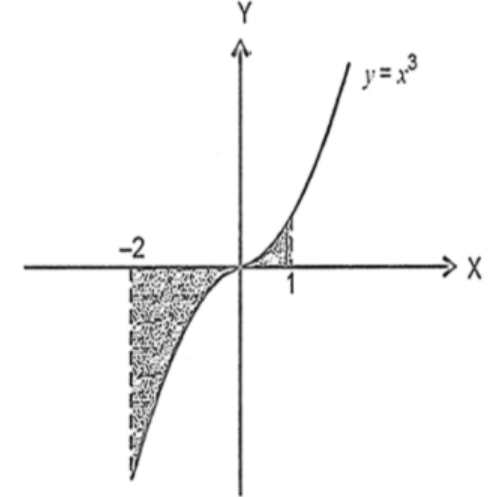The area bounded by the curve $y = {x^3}$, the $X$- axis and the ordinates $x = - 2$ and $x = 1$ isVerified
119.7k+ views
Hint: Firstly, draw the curve $y = {x^3}$ and the ordinates $x = - 2$ and $x = 1$ in the $XY$ plane, then the portion between the curve, the given two ordinates and the $X$-axis are shaded. After that find the area of the shaded portion by using the integration method over the limits of integration.

Complete step-by-step solution:
It is given that we have to find the area bounded by the curve $y = {x^3}$, the $X$- axis and the ordinates $x = - 2$ and $x = 1$.
First of all, draw the curve $y = {x^3}$ and the ordinary $x = - 2$ and $x = 1$. Then highlight the bounded portion between the curve and the ordinates. Which is shown in the figure.Now, calculate the area of the bounded figure by breaking the curve in two parts: the first part is the curve between $x = - 2$ and $x = 0$, and the second part is the curve between $x = 0$ and $x = 1$.
We have studied that the area bounded by the curve and $X$- axis is given by $\left| {\int_a^b {ydx} } \right|$.
Given ordinates bound the curve on the left and right side which gives the upper and lower limits of the integration.
Now, the area of bounded figure is given by $\left| {\int_{ - 2}^0 {{x^3}dx} } \right| + \left| {\int_0^1 {{x^3}dx} } \right|$.
We know that the integration of $\int_a^b {{x^n}} = \left( {\dfrac{{{x^{n + 1}}}}{{n + 1}}} \right)_a^b$ so,
$\Rightarrow$Area $= \left| {\left( {\dfrac{{{x^{3 + 1}}}}{4}} \right)_{ - 2}^0} \right| + \left| {\left( {\dfrac{{{x^{3 + 1}}}}{4}} \right)_0^1} \right|$
$\Rightarrow$Area $= \left| {\left( {\dfrac{{{x^4}}}{4}} \right)_{ - 2}^0} \right| + \left| {\left( {\dfrac{{{x^4}}}{4}} \right)_0^1} \right|$
Now, put the value of upper and lower units in the above integration
$\Rightarrow$Area $= \left| {\dfrac{{{0^4} - {{\left( { - 2} \right)}^4}}}{4}} \right| + \left| {\dfrac{{{1^4} - {0^4}}}{4}} \right|$
$\Rightarrow$Area $= \left| {\dfrac{{0 - 16}}{4}} \right| + \left| {\dfrac{{1 - 0}}{4}} \right|$
$\Rightarrow$Area $= \left| {\dfrac{{ - 16}}{4}} \right| + \left| {\dfrac{1}{4}} \right|$
$\Rightarrow$Area $= \left| { - 4} \right| + \left| {\dfrac{1}{4}} \right|$
Modulus of any number is either positive or negative so,
$\Rightarrow$Area $= 4 + \dfrac{1}{4}$
$\Rightarrow$Area $= \dfrac{{17}}{4}$

Thus, the area bounded by the given curves is $\dfrac{{17}}{4}$ square units.

Note: We need to integrate the in two parts because if we integrate the curve below $X$- axis and above the $X$- axis then the negative value and positive value cancel each other and we will not get the actual area.
Modulus of the integration must be taken while calculating the area because the integration may be negative but area can never be negative so, by taking the modulus of integration we make the value positive.